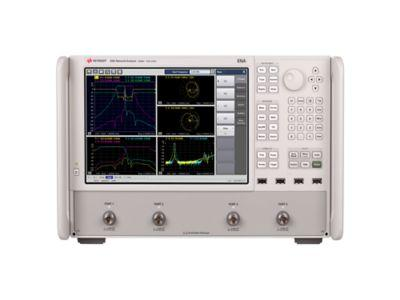PC头# 在使用矢量网络分析仪测量被测器件时，如输入阻抗约为3欧姆，阻抗和回波损耗测量的不确定性是多少？

Q: 在使用矢量 网络分析仪测量被测器件(DUT)时，如输入阻抗约为3欧姆(在2 MHz时)，阻抗和

(S11)测量的不确定性是多少？在这种情况下，阻抗分析仪是否要优于矢量网络分析仪?

A：对于低频和阻抗应用，与使用反射法的矢量网络分析仪（VNA）相比，使用I-V技术的阻抗分析仪能得到更精确的测量结果。常用判定准则是在系统特性阻抗Z0的10倍（或1/10）阻抗范围内，矢量网络分析仪通常能提供足够精度的结果。例如对于Z0= 50Ω的阻抗范围是5Ω至500Ω。但为得到最佳测量精度，DUT阻抗相对Z0的变化应不大于3-5Ω。

ENA 矢量网络分析仪，5 Hz 至 53 GHzZL= 3 Ω (注意: 假定为二端口器件)

S11/回波损耗 (RL) = 1.04 dB

SWR = 16.7 :1

1) 用精密 50Ω 负载端接 DUT 输出，而不是将它接到矢量网络分析仪的传输端口。

2) 如果 DUT 输出被接到矢量网络分析仪的传输端口，在传输端口安装精密衬垫/衰减器，以改进它的“有效”负载匹配。

1) D S11 (dB) = R.L. 测量不确定度 (dB)= -20 log (1 + 0.025)= - 0.214dB ，

-20 log (1 - 0.025)= + 0.22 dB

R.L.测量不确定度 = 1.04 dB, +0.22 dB, -0.214 dB

2) ZL = Z0 (1 + r )/(1- r ) , 这里 ZL = 测量阻抗, Z0 = 50 Ω, r = 反射系数

ZL High = 50 [1 + (-0.887 + 0.025)]/[1 - (-0.887 + 0.025)] = 50 (0.138/1.86) = 3.71 Ω

ZL Low = 50 [1 + (-0.887 - 0.025)]/[1- (-0.887 - 0.025)] = 50 (0.088/1.912) = 2.30 Ω